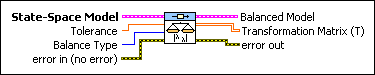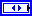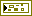# CD Balance State-Space Model (Diagonal) VI

LabVIEW 2018 Control Design and Simulation Module Help

Edition Date: March 2018

Part Number: 371894J-01

»View Product InfoDownload Help (Windows Only)

Owning Palette: State-Space Model Analysis VIs

Requires: Control Design and Simulation Module

Balances the State-Space Model using a diagonal similarity transformation. This transformation reduces the ratios of rows and columns norms of the system matrix A, or of the matrix S defined by the following equation.

S = [A B| C 0]

This VI returns the similarity Transformation Matrix that this VI uses to transform the given system. This VI renames the names of the states using the default names after the transformation.State-Space Model contains a mathematical representation of and information about a system that this VI transforms.Tolerance limits the ill-conditioning of matrix T. This VI estimates this condition by the ratio of maximum and minimum norms of the eigenvalues of T. The default is Inf.Balance Type defines which matrix this VI balances and if this VI allows column/row permutations in the similarity transformation.

 0 A with permutations (default)—This VI considers only matrix A in the calculation of T. This VI can have permutations among rows and columns of matrix A. 1 A without permutations—This VI considers only matrix A in the calculation of T. This VI can not have permutations among rows and columns of matrix A. 2 [A B| C 0]—This VI considers matrix S in the calculation of T. This VI can not have permutations.error in describes error conditions that occur before this node runs. This input provides standard error in functionality.Balanced Model returns the model that results from applying the similarity transformation T to the State-Space Model.Transformation Matrix (T) returns the matrix T that this VI uses to transform the system. The calculation of T is based on LAPACK balance routine.error out contains error information. This output provides standard error out functionality.

## CD Balance State-Space Model (Diagonal) Details

This VI does not support delays unless the delays are part of the mathematical model that represents the dynamic system. To account for the delays in the synthesis of the controller, you must incorporate the delays into the mathematical model of the dynamic system using the CD Convert Delay with Pade Approximation VI (continuous models) or the CD Convert Delay to Poles at Origin VI (discrete models). Refer to the LabVIEW Control Design User Manual for more information about delays and the limitations of Pade Approximation.